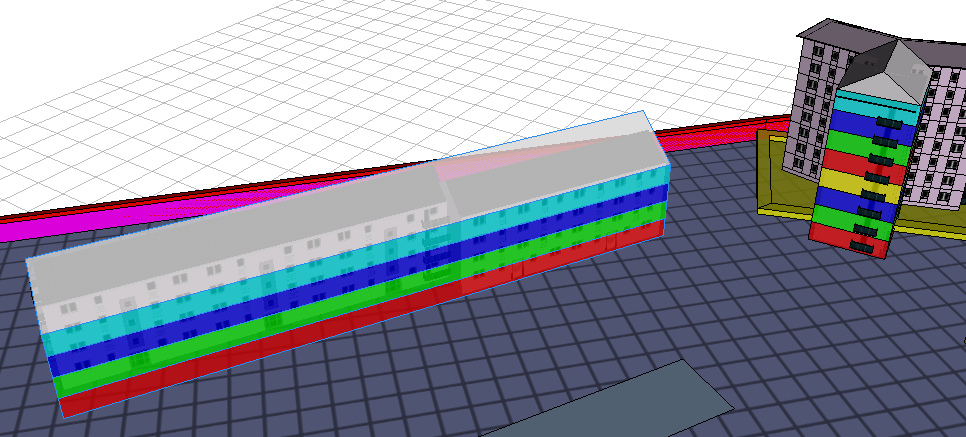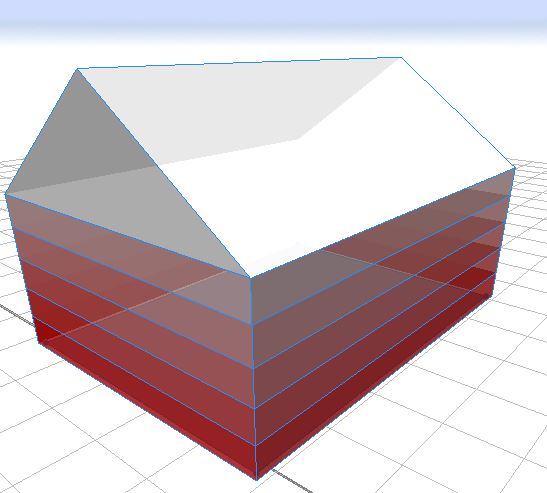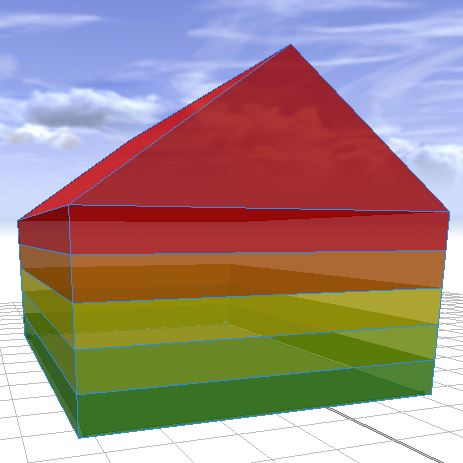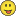# Dynamic case?

4290
7
01-07-2015 01:31 PMby
Regular Contributor

I'm generating some buildings (well, building envelopes) with variable floor counts (attribute "aog") and color for each of the floors. Right now I simply have the maximum number of floors inserted manually and catch the top with "aog-1". Is there a way to do this in a more flexible way?

Here's the rule file:

```version "2014.0"

attr aog = 0
attr maxLength = scope.sx
attr maxWidth = scope.sz
attr maxHeight = 3.0*aog
attr opacityslider = 0.8

#--------------------------------

Lot -->
set(material.opacity,opacityslider)
innerRect
s(maxLength+1,'1,maxWidth+1)
center(xyz)
extrude(maxHeight)
Building

Building -->
comp(f){side: BuildingBody | top: Roof }

BuildingBody -->
split(y){ maxHeight/aog: BuildingFloors }*

BuildingFloors -->
comp(f){all: ColorMe(split.index)}

ColorMe(FloorIndex) -->
case FloorIndex == aog-1: color(0,1,1)
case FloorIndex == 0: color(1,0,0)
case FloorIndex == 1: color(0,1,0)
case FloorIndex == 2: color(0,0,1)
case FloorIndex == 3: color(1,1,0)
case FloorIndex == 4: color(1,0,0)
case FloorIndex == 5: color(0,1,0)
case FloorIndex == 6: color(0,0,1)
case FloorIndex == 7: color(1,1,0)
case FloorIndex == 8: color(1,0,0)
case FloorIndex == 9: color(0,1,0)
case FloorIndex == 10: color(0,0,1)
case FloorIndex == 11: color(1,1,0)
else: color(0.5,0,0)

Roof -->
color(1,1,1)
roofGable(35,0,0,false)```

Example:Tags (3)
1 Solution

Accepted Solutionsby Anonymous User
Not applicable

Here's how to do a gradient (using red, blue, or green). Change your BuildingFloors rule to this, and omit ColorMe rule:

BuildingFloors --> color(1, split.index/(split.total-1), split.index/(split.total-1))

# Change placement of 1 to do the other colors.(G and B)Use two ones to make CMYK colors:

BuildingFloors --> color(1, 1, split.index/(split.total-1))  # Yellow gradient

Or use built in CityEngine colorRamp function:

BuildingFloors --> color(colorRamp("greenToRed", split.index/(split.total-1)))To make roof match, color it using:

color(colorRamp("greenToRed", 1))

Let us know if this this works, then we talk about the rule_ thing.

Chris

7 RepliesRegular Contributor

Hi Z R,
This depends on what you are trying to do. Do the colors have to be the colors you selected? Can they be random colors?

When working with buildings often it helps to develop ranges of color rather than specific floors. So for example I might define that the 1st to 5th floor to be red (FloorIindex<=5), and everything else to be be another color. If you need each floor be a unique color you could just use  color(rand(0,1),rand(0,1), rand(0,1)).

If you need something to be related to the total number of floors, you can also call the split.total to get that total number of splits. I have used this with some basic arithmetic to create automatic ranges and make decisions based on how big or long something actually is. This could for example be used to create a gradient based on the floors starting at one value at the top and descending to another at the bottom.

There are options. I hope this is helpful.

David

David Wasserman, AICPby
Regular Contributor

Hi,

a gradient would be good - the current colors are just for testing. Ideally I'd like to create dynamic rules for each split (basically like rule_splitindex) but that doesn't seem possible. For full-range rand the range doesn't have to be specified, if you want to save some typing. It's also not really random but uses the lot's seed.. which can be set with set(seedian, <somenumber>). Now if only there was some seed that produced gradients..Regular Contributor

Hi ZR,

In that case, it is possible to do this if you are ok with splitting by percentile.

For example lets say we have 10 floors. The split.index tells me what floor my rule is currently evaluating, and the split.total tells me how many total floors there are.

So lets say I want the first 20% to be one color, and the next percentage to be another color.

Pseudo code would be:
attr split_Percent_1=.2

attr split_Percent_2=.4

case split.index/split.total-1<= split_Percent_1: color(1,0,0)

case (split.index/split.total-1)-split_Percent_1<= split_Percent_2: color(1,1,0)

else: color(1,1,1)

This code has errors/problems, but the idea is that you are coloring based on the percent of the total number of floors. If you want something more complex there are other options to try. I am trying to understand the design specifications you have for this split.index based rule.

Kind Regards,

David

David Wasserman, AICPby
Regular Contributor

Thanks,

but I need to use fixed heights (floors*3m), so it's not applicable in this case.

Might come in handy later, though!by Anonymous User
Not applicable

Here's how to do a gradient (using red, blue, or green). Change your BuildingFloors rule to this, and omit ColorMe rule:

BuildingFloors --> color(1, split.index/(split.total-1), split.index/(split.total-1))

# Change placement of 1 to do the other colors.(G and B)Use two ones to make CMYK colors:

BuildingFloors --> color(1, 1, split.index/(split.total-1))  # Yellow gradient

Or use built in CityEngine colorRamp function:

BuildingFloors --> color(colorRamp("greenToRed", split.index/(split.total-1)))To make roof match, color it using:

color(colorRamp("greenToRed", 1))

Let us know if this this works, then we talk about the rule_ thing.

Chrisby
Regular Contributor

That's just what I was looking for. ThanksRegular Contributor

The Gradient is a lot easier to do than a percentile split. This is the best option if this is what you need. Glad Chris could make an example.

David Wasserman, AICP